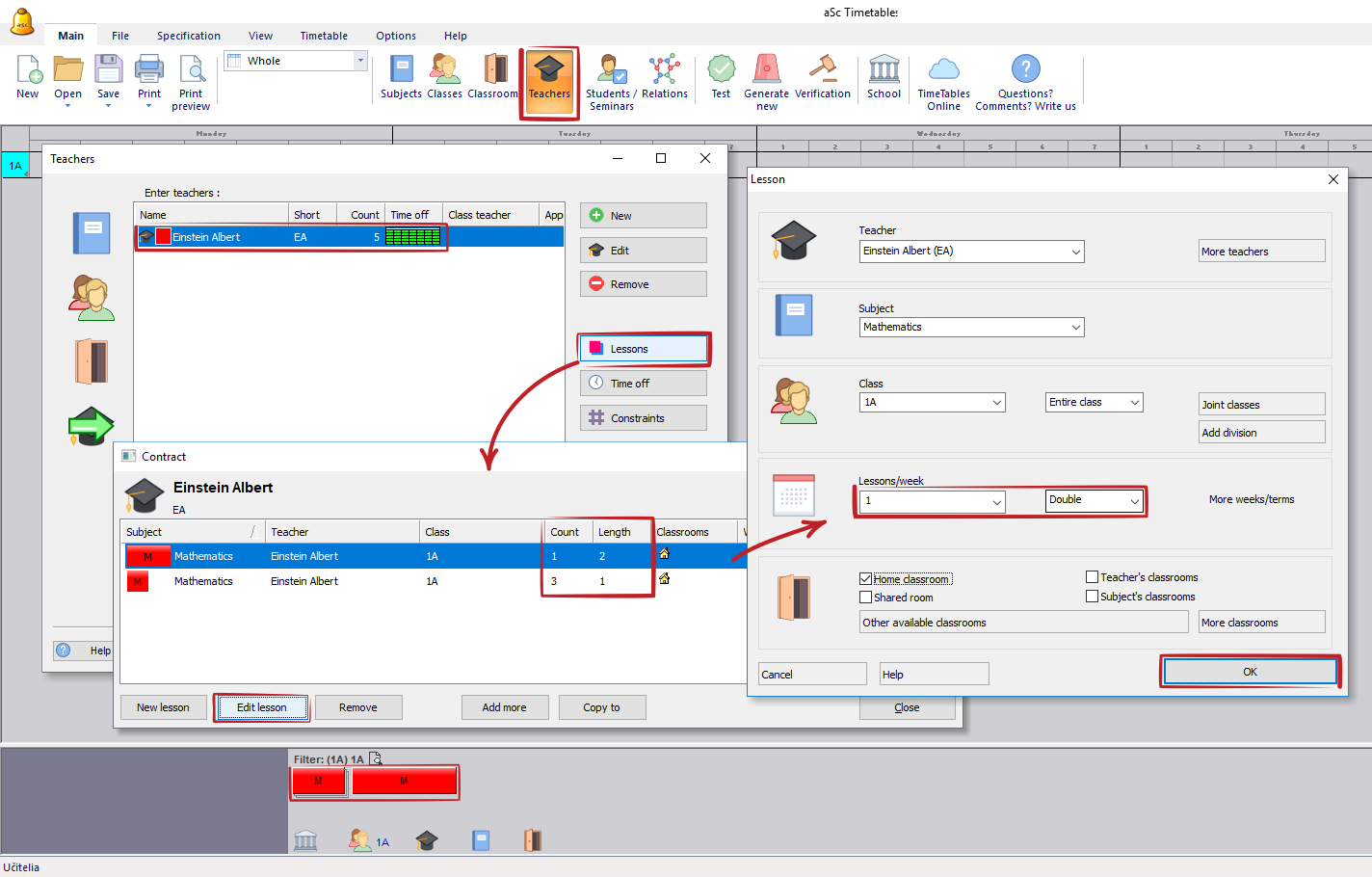aSc TimeTables - Data input - Lessons

I want to have Single AND Double lessons of one subject! E.g 1+1+1+2

You can simply add into teachers' lessons that he is teaching e.g. 3 single and 1 double.It means he is teaching 1+1+1+2 = 5 lessons per week.

Notes: